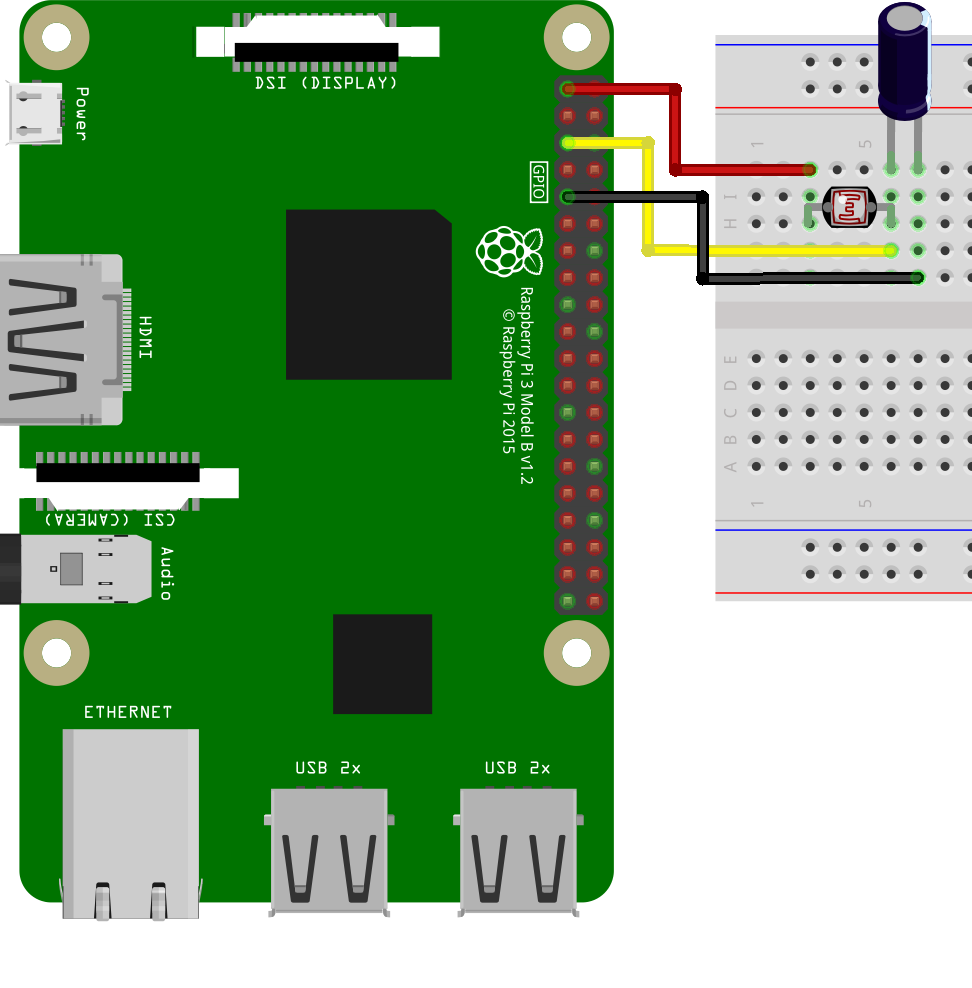## Light intensity measurement with Raspberry Pi 3 LDR

tarafından
6918What is LDR?
LDR is a two-pole switching element used to measure light intensity, which we usually know as a photocell. The resistance value between the two ends changes according to the amount of light falling on them. Thanks to this function we can measure the amount of light.

If you have previously performed a similar application with Arduino, you know that LDR is connected to the analog input. However, our Raspberry Pi does not have an analogue input. As a solution for this situation we have to install an RC circuit.

RC circuit for LDR measurement:
The RC circuitry consists of a capacitor and a resistor. Capacitors are used in circuits for load storage. The current charges the capacitor, which causes its voltage to rise. By limiting the current to the capacitor, the resistor slows down the charging of the capacitor. This means that the time at which the capacitor is filled determines the value of the resistor. The amount of light determines the charging time of the capacitor, because it changes according to the light that falls on the resistor of the LDR. Since our Raspberry Pi recognizes this as a logical 1 level when the capacitor reaches 1.8 V, the time it takes the GPIO pin to reach the logical 1 level gives information about the light level.

Raspberry Pi – LDR RC schematic: